• Call Now

1800-102-2727•

# Integration: Evolution, Definition, Definite and Indefinite Integrals, Standard Formulas, Practice Problems and FAQs

Do you understand how integration developed prior to defining it, its uses, characteristics, and instances from real life? If so, that's fantastic; if not, this piece is all about a fundamental comprehension of integration.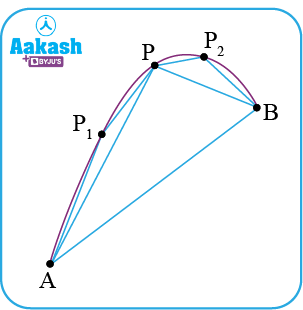All credit goes to Archimedes, who discovered the region enclosed by a parabola and made numerous triangles from it. Archimedes discovered the area under a parabolic arc is 43 of the area of a triangle inscribed into that arc.

As in the figure, ar(arc PP2B)=4/3 ar( Δ PP2B). He used several known properties of triangles and segments, which led to the construction of the method of exhaustion. Here, method of exhaustion means trying to find out the area of a shape by inscribing the polygons. But Archimedes didn't apply ideas like limits and convergence. Archimedes was therefore both incredibly close to and extremely far from discovering the integral.

All of the geometers made the same claim. Fill a geometric shape with a significant but limited number of polygons. The area of the figure would be equal to the sum of the areas of these polygons. They did not, however, view this as a series.

Based on exhaustion Isaac Newton and Gottfried Wilhelm Leibniz independently developed the principles of integration. They believed that the area under a curve was composed of an infinite number of rectangles of infinitesimal width. Later, Bernhard Riemann provided a precise definition of integrals that is based on a limiting process that divides a curved region into infinitesimally thin vertical slabs in order to approximate the area of the region. Let’s understand some more interesting things about integration through this article.

• Definition of integration
• Integration as an Inverse Process of Differentiation
• Application of Integration
• Definite Integrals
• Indefinite Integrals
• Standard Integration Formulas
• Practice Problems

## Definition of Integration

Let us take a function f which is differentiable for all possible values of interval I i.e. Df(x) can be found out at each and every point of I. Now if we try to think in reverse manner if Df(x) is known at the beginning for every possible values in the interval I, can we evaluate the function f(x)? Let’s understand this with the example below.

Let g(x) be the derivative of  f(x) i.e. g(x) = Df(x), here f(x) is called as antiderivative (or primitive) of g(x). Further, all these anti derivatives can be given by a formula which is known as indefinite integral i.e. ∫g(x)dx = f(x), where ∫ is the symbol of integration. This process of finding out the antiderivatives is known as integration.

∫g(x)dx is read as integration of g(x) with respect to x.

## Integration as an Inverse Process of Differentiation

Instead of differentiating a function, we are asked to evaluate the original function, using the derivative of the function. Let's think about the following example:

We are aware that ax ln a is the differentiation of ax.

It is represented mathematically as:

The derivative of ax in this case is ax ln a. Therefore, the antiderivative of ax ln a is ax. Additionally, every real number "k" is regarded as a constant function, and its derivative is zero.

Equation (1) can therefore be expressed as

Where "k" stands for the integration constant or arbitrarily chosen constant.

In general, the function can be written as follows:

. Where x belongs to the interval I,

As a result, the antiderivative of a function (Integration) is symbolically represented as follows:

∫ g(x) dx = f(x) + k

## Application of Integration:

There are several instances of the integration process in both theoretical and practical contexts. The efforts to solve the following kinds of problems led to the development of integral calculus:

(A) the issue of evaluating a function whenever its derivative is known;
(B) the issue of calculating the region enclosed by a function's graph under specific circumstances.

These two issues give rise to the two integral types:

1. Definite Integrals and
2. Indefinite integrals

These two collectively make up the integral calculus.

## Definite integrals:

Definite integral is represented as ${\int }_{a}^{b}f\left(x\right)dx$, in which a is called lower limit and b is called the upper limit of the integration. And it represents the area bounded by the curve f(x), the ordinates x = a. x = b and x - axis. Let us discuss more about this with the help of below graph: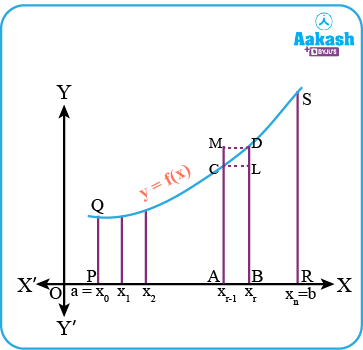Let us think in a manner that the interval a to b is divided into n equal intervals as xo to x1, x1 to x2, x2 to x3 ,

where ${x}_{0}=a,{x}_{1}=a+h,.....{x}_{r}=a+rhand{x}_{n}=b=a+nh;$ hence $n=\frac{b-a}{h}$.

Now, The area under the curve can be found out by summing up the area of small regions (for example ACDLB) lying between intervals. Also by observation we can conclude that ar(ACLB) < ar(ACDLB) < ar(AMDB). Here if we take large number of the intervals i.e. n∞, or h0 then all three areas ACLB, ACDLB and AMDB will be almost equal to each other.

Therefore, we can form the following sums for the area of lower rectangles and upper rectangles.

S= $h\left[f\left({x}_{o}\right)+f\left({x}_{1}\right)+...............+f\left({x}_{n-1}\right)\right]$ (for lower rectangles)
S= $h\left[f\left({x}_{1}\right)+f\left({x}_{2}\right)+...............+f\left({x}_{n}\right)\right]$ (for upper rectangles)

Hence, S1 = $h{\sum }_{0}^{n-1}f\left({x}_{r}\right)$ and S= $h{\sum }_{1}^{n}f\left({x}_{r}\right)$

Here if we conclude the discussion made above we can come up with conclusion that;
Area of region PQSRP;

We know that the required area will be between the summation of the area of rectangles above the curve and the summation of the area of rectangles below the curve. So in order to avoid the computational difficulty we can assume a rectangle with the height equal to that of the curve at the left hand edge of each interval. Thus rewriting the area of region PQSRP.

${\int }_{a}^{b}f\left(x\right)dx=$$\underset{h\to 0}{lim}h\left[f\left(a\right)+f\left(a+h\right)+........+f\left(a+\left(n-1\right)h\right)\right]$

## Indefinite integrals:

As y = x+ k; represents the family of the curves where k is an arbitrary constant similarly ${\int }_{a}^{b}f\left(x\right)dx=g\left(x\right)+c;$ represents the family of the integrals. With different values of c we can get all the members of the family, and these members together are called indefinite integrals.

Let us try to visualise y = x3+k by means of graphical representation for different values of k as k = -2, -1, 0, 1 and 2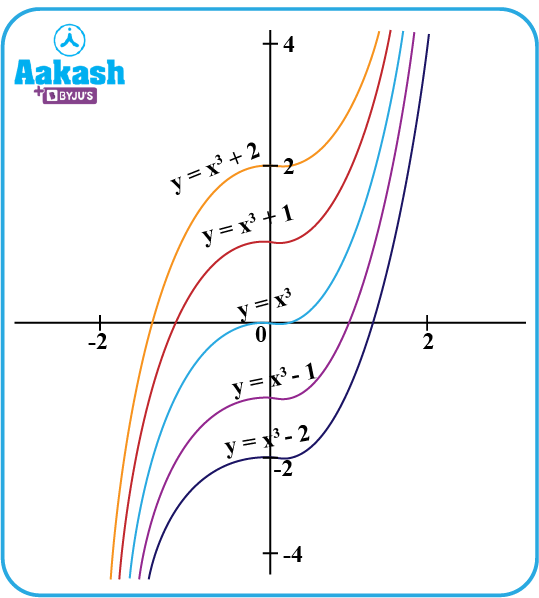## Standard Integration Formulas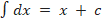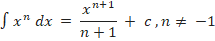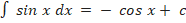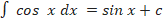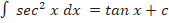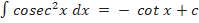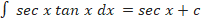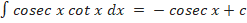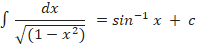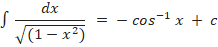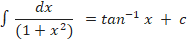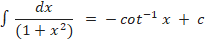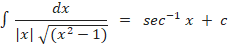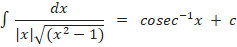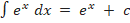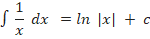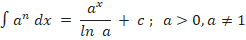## Practice Problems

Example 1: Find the integral of the function:

Solution: we have

x3-1x2 dx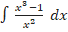= (x1+11+1 + c1) - (x-2+1-2+1 + c2)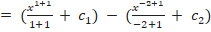∫x3-1x2 dx= x22 + 1xc ;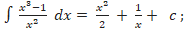where c = c1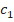-c2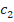is constant of integration

Example 2: Find the integral of the function: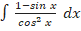Solution: we have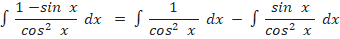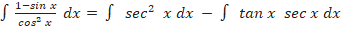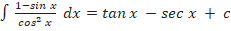Example 3: Find the integral of the function: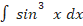Solution: We have,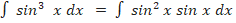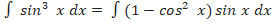Let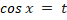so that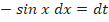therefore ,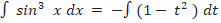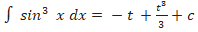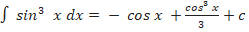Example 4: Find the integral of the function: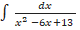Solution: we have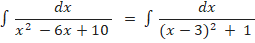Let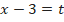so that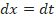therefore ,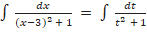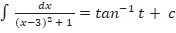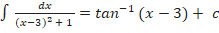## Frequently Asked Questions on Integration

Question 1: Can we discuss both integration and differentiation at a point?
No, At some point, we can discuss the derivative. We talk about integration over an interval.

Question 2: Are integral and integrands the same?
No, ∫f(x) dx is integral of f with respect to x, while only f(x)is integrand in ∫f(x) dx.

Question 3: How is integration useful in real life situations?
On a tiny scale Integration is useful for determining the centre of mass and gravity, and on a large scale, it aids in resolving intriguing issues from a variety of fields, including probability, finance, and economics.

Question 4: How to choose the substitution while solving integration problems?
Typically, we substitute a function whose derivative also appears in the integrand.

Question 5: Explain one real life use of the derivation and integration.
When the distance travelled at any given time t is known, the derivative can be used to determine various physical quantities, such as the speed and acceleration of the moving particle. Similar to this, when the velocity at time t is known, the integral is utilised to determine the displacement.Talk to our expert
Resend OTP Timer =
By submitting up, I agree to receive all the Whatsapp communication on my registered number and Aakash terms and conditions and privacy policy